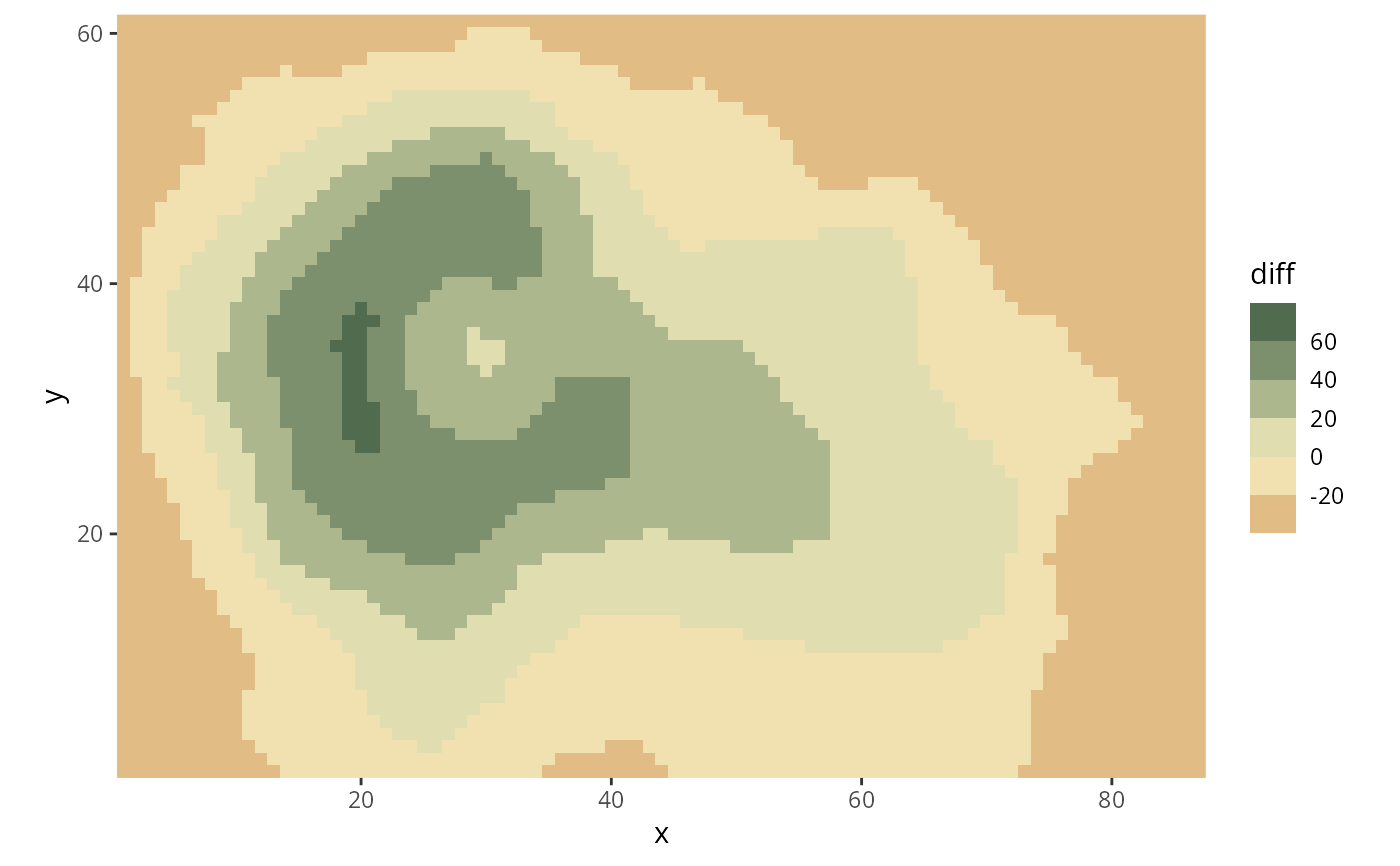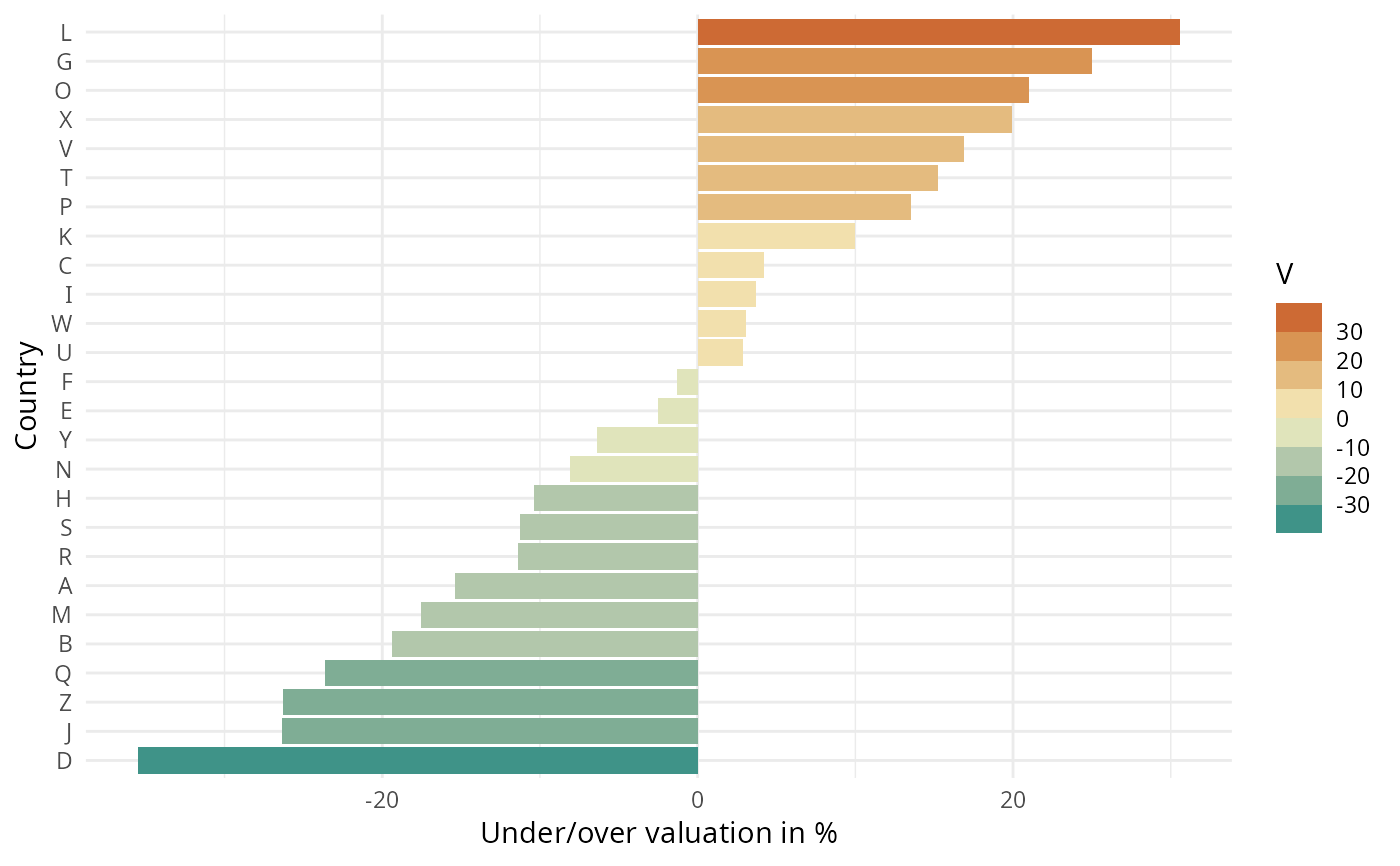Binned ggplot2 color scales using the color palettes generated by divergingx_hcl.

scale_colour_binned_divergingx(
palette = "Geyser",
c1 = NULL,
c2 = NULL,
c3 = NULL,
l1 = NULL,
l2 = NULL,
l3 = NULL,
h1 = NULL,
h2 = NULL,
h3 = NULL,
p1 = NULL,
p2 = NULL,
p3 = NULL,
p4 = NULL,
cmax1 = NULL,
cmax2 = NULL,
alpha = 1,
rev = FALSE,
mid = 0,
na.value = "grey50",
guide = "coloursteps",
n_interp = 11,
aesthetics = "colour",
...
)

scale_color_binned_divergingx(
palette = "Geyser",
c1 = NULL,
c2 = NULL,
c3 = NULL,
l1 = NULL,
l2 = NULL,
l3 = NULL,
h1 = NULL,
h2 = NULL,
h3 = NULL,
p1 = NULL,
p2 = NULL,
p3 = NULL,
p4 = NULL,
cmax1 = NULL,
cmax2 = NULL,
alpha = 1,
rev = FALSE,
mid = 0,
na.value = "grey50",
guide = "coloursteps",
n_interp = 11,
aesthetics = "colour",
...
)

scale_fill_binned_divergingx(..., aesthetics = "fill")

## Arguments

palette

The name of the palette to be used.

h1, h2, h3, c1, c2, c3, l1, l2, l3, p1, p2, p3, p4, cmax1, cmax2

Parameters to customize the scale. See divergingx_hcl for details.

alpha

Numeric vector of values in the range [0, 1] for alpha transparency channel (0 means transparent and 1 means opaque).

rev

If TRUE, reverses the order of the colors in the color scale.

mid

Data value that should be mapped to the mid-point of the diverging color scale.

na.value

Color to be used for missing data points.

guide

Type of legend. Use "coloursteps" for color bar with discrete steps.

n_interp

Number of discrete colors that should be used to interpolate the binned color scale. For diverging scales, it is important to use an odd number to capture the color at the midpoint.

aesthetics

The ggplot2 aesthetics to which this scale should be applied.

...

common binned scale parameters: name, breaks, labels, and limits. See binned_scale for more details.

## Details

Available CARTO palettes: ArmyRose, Earth, Fall, Geyser, TealRose, Temps, Tropic.

Available ColorBrewer.org palettes: Spectral, PuOr, RdYlGn, RdYlBu, RdGy, BrBG, PiYG, PRGn, RdBu.

If both a valid palette name and palette parameters are provided then the provided palette parameters overwrite the parameters in the named palette. This enables easy customization of named palettes.

## Examples

library("ggplot2")

# volcano plot (difference from mean height)
nx = 87
ny = 61
df <- data.frame(diff = c(volcano) - mean(volcano), x = rep(1:nx, ny), y = rep(1:ny, each = nx))
ggplot(df, aes(x, y, fill=diff)) +
geom_raster() + scale_fill_binned_divergingx(palette = "Fall", rev = TRUE) +
coord_fixed(expand = FALSE)# adapted from stackoverflow: https://stackoverflow.com/a/20127706/4975218

# generate dataset and base plot
set.seed(100)
df <- data.frame(country = LETTERS, V = runif(26, -40, 40))
df$country = factor(LETTERS, LETTERS[order(df$V)]) # reorder factors
gg <- ggplot(df, aes(x = country, y = V, fill = V)) +
geom_bar(stat = "identity") +
labs(y = "Under/over valuation in %", x = "Country") +
coord_flip() + theme_minimal()

# plot with diverging scale "Geyser"
gg + scale_fill_binned_divergingx(palette = "Geyser",  n.breaks = 6)